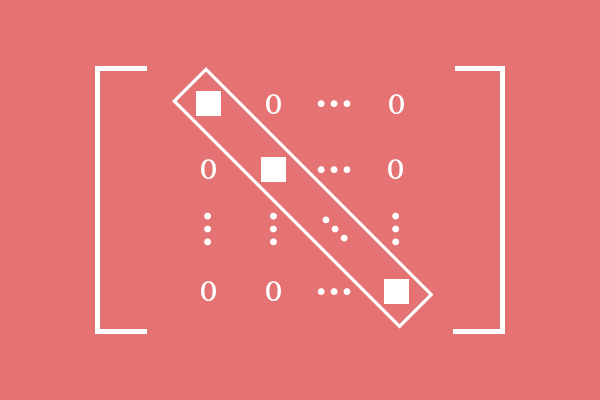Scalar Matrix

Definition

A diagonal matrix whose diagonal elements are equal, is called a scalar matrix.Scalar matrix is a square matrix and the number of rows is equal to the number of columns in it. So, a diagonal matrix is possible by the square matrix.

A diagonal matrix contains elements only on the leading diagonal and the remaining elements are zeros. In the same pattern, scalar matrices contain elements in principal diagonal but those elements are same.

$M = \begin{bmatrix} e_{11} & 0 & 0 & \cdots & 0\\ 0 & e_{22} & 0 & \cdots & 0\\ 0 & 0 & e_{33} & \cdots & 0\\ \vdots & \vdots & \vdots & \ddots & \vdots\\ 0 & 0 & & \cdots & e_{mm}\\ \end{bmatrix}$

Therefore, the diagonal elements are same and the non-diagonal elements are zero in scalar matrices.

$e_{11} = e_{22} = e_{33} = \cdots = e_{mm}$

Example

$A = \begin{bmatrix} 2 & 0 \\ 0 & 2 \end{bmatrix}$

$A$ is a scalar matrix of order $2$. In this matrix, the leading diagonal elements are same and it is $2$ but the non-diagonal elements are zero.

$B = \begin{bmatrix} -6 & 0 & 0 \\ 0 & -6 & 0 \\ 0 & 0 & -6 \\ \end{bmatrix}$

$B$ is a diagonal matrix basically but it is having same element in its diagonal and the element is $-6$. Hence, the square matrix $B$ is known as a scalar matrix of order $3$.

$C = \begin{bmatrix} 7 & 0 & 0 & 0 \\ 0 & 7 & 0 & 0 \\ 0 & 0 & 7 & 0 \\ 0 & 0 & 0 & 7 \end{bmatrix}$

In square matrix $C$, nonzero element $7$ is arranged on principal diagonal but the other elements are nonzero elements as non-diagonal elements. Therefore, the square matrix $C$ is identified as a diagonal matrix and also as a scalar matrix.

Latest Math Problems
Email subscription
Math Doubts is a best place to learn mathematics and from basics to advanced scientific level for students, teachers and researchers. Know more